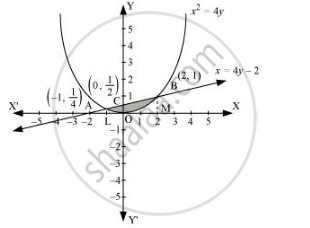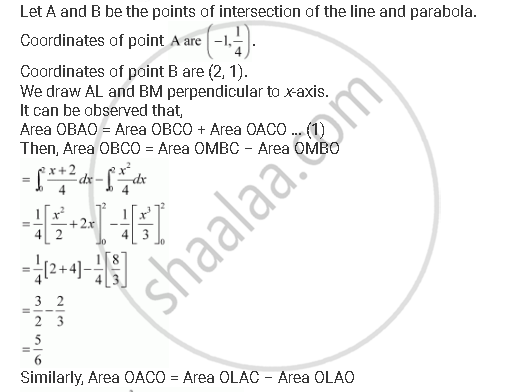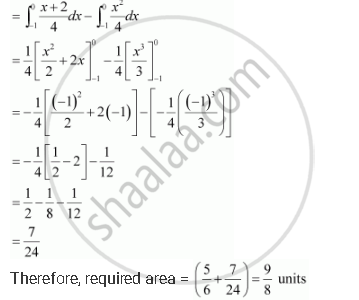Share

# Find the Area Bounded by the Curve X2 = 4y and the Line X = 4y – 2 - CBSE (Commerce) Class 12 - Mathematics

#### Question

Find the area bounded by the curve x2 = 4y and the line x = 4– 2

#### Solution

The area bounded by the curve, x2 = 4y, and line, x = 4– 2, is represented by the shaded area OBAO.Is there an error in this question or solution?

#### APPEARS IN

NCERT Solution for Mathematics Textbook for Class 12 (2018 to Current)
Chapter 8: Application of Integrals
Q: 10 | Page no. 366
Solution Find the Area Bounded by the Curve X2 = 4y and the Line X = 4y – 2 Concept: Area Under Simple Curves.
S